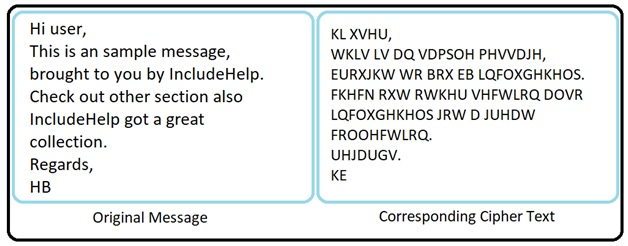# Cryptography: Caesar Cipher and Its Python Implementations

In this tutorial, we will learn about the introduction of the basic concept in cryptography and discusses the Caesar Cipher and its Python implementation. By Himanshu Bhatt Last updated : May 24, 2023

Before we start let's some basic terminology...

The art and science to achieve security by encoding messages to make them unreadable are known as Cryptography. That's what the whole article is going about.

The technique to decode an unreadable code to readable again without knowing how they were initially converted from readable to unreadable is Cryptanalysis. That's what we'll do in our later articles.

Thus, Cryptology = Cryptography + Cryptanalysis.

Cryptography is used since ages performed by manual techniques but the basic framework has always remained less or more the same, definitely, there were a lot of improvements. While this article is all theory but no need be disheartened we'll cover them too.

We have two types of text:

1. Plain (or clear) text: Which is an actual message that both sender and receiver can understand also by anyone else who gets an access to that message.
2. Cipher text: When any plain text is codified using a suitable scheme and the resulting message is a cipher text.

There are two ways by which we can primarily change plain text to cipher text by Substitution and Transposition.

## Caesar Cipher

This Scheme was first proposed by Julius Caesar, cryptography is used since that time.

In this Substitution cipher technique, each character of the plaintext message will be replaced by another character, symbol or number.

Caesar cipher is another example of a substitution cipher where it replaces each alphabet from the message to an alphabet 3 places down the line.### Caesar Cipher: Python Encoding

```string = input("Enter a string\n")
string= str.upper(string)
for x in string:
if(x==' '):
print(' ',end='')
elif(ord(x)-ord('A')+3 >= 26 ):
print(chr(ord(x)-26+3), end='')
else:
print (chr(ord(x)+3), end='')
```

### Caesar Cipher: Python Decoding

```string = input('Enter Decode text: ')
string = str.upper(string)

for x in string:
if(x==' '):
print(' ',end='')
elif(ord(x)-ord('A')-3<0):
print(chr(ord(x)-3+26), end='')
else:
print(chr(ord(x)-3), end='')
```

Just to make an attacker's life more difficult we generalized the Caesar Cipher by not necessarily change original alphabet by a third place down the line but instead it can be any place down the line.

## Modified Version of Caesar Cipher

Just to make an attacker's life more difficult we generalized the Caesar Cipher by not necessarily change original alphabet by a third place down the line but instead it can be any place down the line.

In modified Version, and alphabet can be changed with any other alphabet but once the replacement scheme is decided then it would be constant and will use for all other alphabets in that message.

Since English has 26 alphabets then there are 25 possible replacement schemes (replacement of an alphabet with itself is senseless).

Example:

RWLUDMNQNUY RB JFNBXVN

To change above Cipher Text into the plain text we need to use brute-force (trying all available options) thus we got 25 results.

```    1.	QVKTCLMPMTX QA IEMAWUM
2.	PUJSBKLOLSW PZ HDLZVTL
3.	OTIRAJKNKRV OY GCKYUSK
4.	NSHQZIJMJQU NX FBJXTRJ
5.	MRGPYHILIPT MW EAIWSQI
6.	LQFOXGHKHOS LV DZHVRPH
7.	KPENWFGJGNR KU CYGUQOG
8.	JODMVEFIFMQ JT BXFTPNF
9.	INCLUDEHELP IS AWESOME
10.	HMBKTCDGDKO HR ZVDRNLD
11.	GLAJSBCFCJN GQ YUCQMKC
12.	FKZIRABEBIM FP XTBPLJB
14.	DIXGPYZCZGK DN VRZNJHZ
15.	CHWFOXYBYFJ CM UQYMIGY
16.	BGVENWXAXEI BL TPXLHFX
17.	AFUDMVWZWDH AK SOWKGEW
18.	ZETCLUVYVCG ZJ RNVJFDV
19.	YDSBKTUXUBF YI QMUIECU
20.	XCRAJSTWTAE XH PLTHDBT
21.	WBQZIRSVSZD WG OKSGCAS
22.	VAPYHQRURYC VF NJRFBZR
23.	UZOXGPQTQXB UE MIQEAYQ
24.	TYNWFOPSPWA TD LHPDZXP
25.	SXMVENOROVZ SC KGOCYWO
```

Here we tried all possible outcomes and the 9th one was our message.

### A modified version of Caesar Encoding

```string = input('Enter Input: ')
key = int(input('Enter a KEY (1-25): '))
string= str.upper(string)
for x in string:
if(x==' '):
print(' ',end='')
elif(ord(x)-ord('A')+key >= 26 ):
print(chr(ord(x)-26+key), end='')
else:
print (chr(ord(x)+key), end='')
```

### A modified version of Caesar Decoding

```string = input('Enter Decode text: ')
string = str.upper(string)

for key in range(1,26):
for x in string:
if(x==' '):
print(' ',end='')
elif(ord(x)-ord('A')-key<0):
print(chr(ord(x)-key+26), end='')
else:
print(chr(ord(x)-key), end='')
print(' ')
```